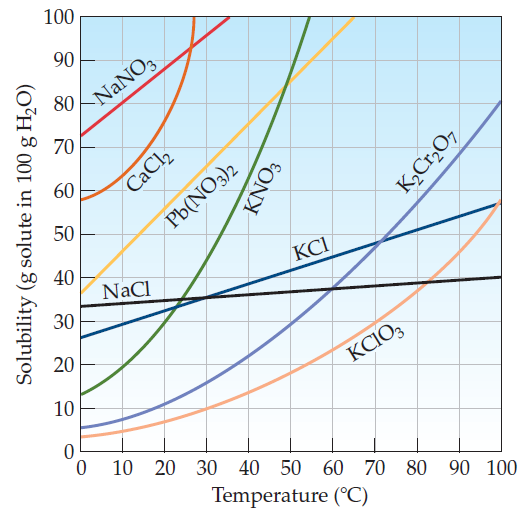×
Get Full Access to Introductory Chemistry - 5 Edition - Chapter 13 - Problem 34p
Get Full Access to Introductory Chemistry - 5 Edition - Chapter 13 - Problem 34p

×

A KCl solution containing 42 g of KCl per 100 g of water is cooled from 60 °C to 0 °CISBN: 9780321910295 34

Solution for problem 34P Chapter 13

Introductory Chemistry | 5th Edition

• Textbook Solutions
• 2901 Step-by-step solutions solved by professors and subject experts
• Get 24/7 help from StudySoup virtual teaching assistantsIntroductory Chemistry | 5th Edition

4 5 1 309 Reviews
25
0
Problem 34P

A KCl solution containing 42 g of KCl per 100 g of water is cooled from 60 °C to 0 °C. What happens during cooling? (See Figure 13.4.)

Figure 13.4 Solubility of some ionic solids as a function of temperatureStep-by-Step Solution:
Step 1 of 3

Solution 34P:

The solubility of a solid in a solution is dependent on temperature. Thus the solubility of most solids in water increases with increasing temperature.

From the figure 1., it is observed the solubility of  KCl at 60 °C  is 45 g per 100 g of water whereas the solubility of  KCl at 0 °C  is 28 g per 100 g of water. Thus, a KCl solution containing 42 g of KCl per 100 g of water is cooled at 60 °C  forms unsaturated solution.

Therefore, the difference between the amount of KCl  which could be dissolved at 60∘C and the amount of KCl which could be dissolved at 0∘C will precipitate out of solution.

Thus, when the KCl solution having 42 g of KCl per 100 g of water cools from from 60 °C to 0 °C, the solution becomes supersaturated and the excess solid precipitates out of solution. When the solution cools slowly,it forms crystals as it comes out of solution.

Step 2 of 3

Step 3 of 3

ISBN: 9780321910295

Unlock Textbook Solution